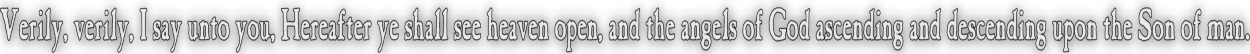None:
Polyps:
Strongs:MetaphysicsEquivalence Revisited In a prior page we defined an equivalence relation '~' to relate x~y if and only if x in "conceiving" of a being y, x would do so upon the assumption that y could likewise reciprocate. I.e. "being" is a term restricted to those beings x and y that may be imagined to exist as we do, i.e. with consciousness that could in their essence also understand that we as 'x' are also a "being". So God may universally conceive of all of us, 'x', if and only if we were able to conceive of His existence as necessary. Likewise I can only conceive of a being y that would understand my own contingency of existence. Clearly this statement of reciprocation implies in itself transitivity and symmetry, (plus it is consistently reflexive) so we have an equivalence relation. i.e. x~x (reflexivity) x~y => y~x (Symmetry) x~y and y~z => x~z (Transitivity) We stated that if a being can conceive of it's own existence it should by the nature of its contingency or necessity likewise be able to conceive of a necessarily existent being. i.e. y~y => P(y~N(G)) which by nature of the definition of the relation, implies N(G~y) Of course, if x may not conceive of a being 'y', then G is unable to do so either, so !P(x~y) => !P(G~y) We assume this since G is perfect. So "x" must belong to some other equivalence class than 'y'. Yet there is only one class since N(G~x) for all x. Likewise if for some arbitrary y we have ¬P(G~y) then ¬P(y~G) thus ¬P(y~y) (y can not conceive of itself, very strange!) Thus it is possible to conceive of a "being" God. However we have trouble with our notation in using '~' as our relation (We had to use ¬ for logical not above). We will introduce another operator cogx(y) for x~y which will allow us some greater ability to say cogx(¬y) or "x can conceive of the non-existence of y." Then it becomes true that cogG(¬G) is false if G is a necessary being. (But cogx(¬x) is an ability of x if x is also contingently existent.) We then easily recover the terms ¬P(cogx(y)) and N(cogx(y)) etc. So to revisit the result of our equivalence relation, if cogx(y) <=> cogy(x) then we may state or rather posit that P(cogx(¬x)) => P(cogx(G)) i.e. a contingently existent being may possibly conceive of a necessary being. But then we have a contradiction on the modus tollens, for: ¬P(cogx(G)) => ¬P(cogx(¬x)) or ¬(cogx(G)) => ¬(cogx(¬x)) Or, that 'x' can not consider itself to be contingent and has therefore conceived itself as necessary! So we have a contradiction; yet this contradiction, is it "necessary existence" for God that is contradicted? Or is it true that x can from the existence of God perceive of himself as eternal, in the perception of the "eternal soul" of 'x'? More to the point, is it impossible to ascend such a "chain in time" through a poset of contingently existent beings to arrive at the necessary with the infinite? Thus I mean, that every being, were they to imagine "someone else", existing for a year more than they and so on ad infinitum - then would they ever conceive of the truly necessary? Is such an eternal idea or conception itself necessary through the axiom of choice? (We then arrive at a statement that necessary existence is some form of predicate. It can not be contingent) and that God, if He truly exists and even if the modus ponens above is false, then God exists necessarily. That stated; the distinction made between existence as a predicate (or not) is equivalent to the selection or ascension of the poset from the axiom of choice (Zorn's lemma) and therefore is demonstrably shown to be as true as any other construction, it merely being axiomatic. (And hence a predicate of logic, as well founded as any other.) That stated, necessary existence is then justly conceivable - and the modus ponens seems well founded. That is, at least the idea that if "necessity of being" is also essence to that idea of perfection ascribed to as "God" Then God is rationally conceived to exist. The modus tollens then, i.e. that the inability to rationally conceive of the necessary, may also be argued to merely reduce in subject to the "immortal" amongst the contingent - (yet, that is not the sense implied by the ascendence of the poset.) Thus, as one did not choose the contingent, but the necessary (Hence the clear distinction of predicate) we find the result that if nothing contingent can conceive of its own non-existence rationally, then "I think therefore I am" as it is put, reduces to in part; "I can not distinguish my non-existence from my thought." Either way, were we to assume that the tables are reversed, we would have another contradiction. if P(cogG(¬G)) => ¬P(cogG(¬x)) Now the left hand side is by Anselm's argument a "nonsense" but if God understands contingent existence, He may reason in our place and do so perfectly: Then, upon His own necessary existence He will find that the modus ponens above that x may not be considered contingent (by itself) is a actually a fact for all under God. i.e. P(cogG(¬G)) => ¬P(cogG(¬x)) So, as God is consistent we must have the latter but not the former. i.e. N¬(cogG(¬x)) Thus to God, all are alive. We need some mechanism where God may reason as if He were in our place, understanding our nature and reasoning from our own contingency. I.e. ¬P(cogG(¬x)) is a nonsense surely? Maybe Not! Continue To Next Page Return To Section Start'